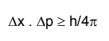# Heisenberg’s Uncertainty Principle

All moving objects eg. car, ball, etc thrown in the air move along definite paths. Hence their position and velocity can be measured accurately at any instant of time.

As a cause of dual nature of matter, Heisenberg, gave a principle about the uncertainties in simultaneous measurement of position and momentum (mass x velocity) of small particles.

AS per this principle “It is impossible to measure simultaneously the position and momentum of a small microscopic moving particle with absolute accuracy or certainty” means, if an attempt is made to measure any one of these two quantities with higher accuracy, the other becomes less accurate.

The product of the uncertainty in position (x) and the uncertainty in the momentum (p) is equal to or greater than h/4 where h is the Planck’s constant.

p = m.v

where m is the mass of the particle and

v is the uncertainty in velocity

Thus, the mathematical expression for Heisenberg’s uncertainty principle is written asPost By : Ramashankar Rajppot 18 Jan, 2020 3297 views Chemistry